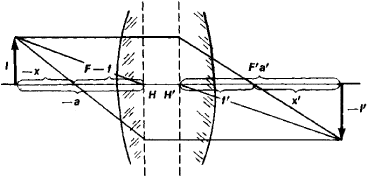Linear Magnification

Also found in: Dictionary, Thesaurus, Medical, Legal.
Related to Linear Magnification: Angular magnification

Linear Magnification

a numerical quantity that determines the scale of the image produced by an objective. It is equal to the ratio of the lengths l and l’ of segments that are perpendicular to the optical axis of the objective and lie in contiguous planes of the image and object, respectively. For an ideal objective—that is, an objective free of aberrations—the linear magnification is

β = I’/l = a’/a = (f’ – a’)/f’ = f/(fa) = –(x’/f’) = –(f/x)

where the symbols are those used in Figure 1. When β > 0 the image is erect, and when β < 0 it is inverted. The linear magnification of objectives of telescopes and field glasses is extremely small, but in the objectives of microscopes it may reach 100.Figure 1.

Site: Follow: Share:
Open / Close